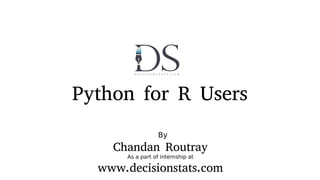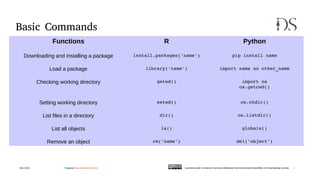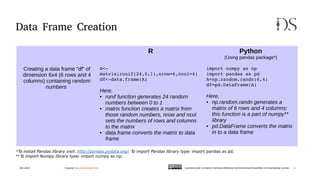Successfully reported this slideshow.

# Python for R Users

266

Share×
1 of 34
1 of 34

# Python for R Users

266

Share

A side by side comparison of using Python for R users using a standard data science/ analytics workflow

A side by side comparison of using Python for R users using a standard data science/ analytics workflow

## More Related Content

### Related Books

Free with a 30 day trial from Scribd

See all

### Related Audiobooks

Free with a 30 day trial from Scribd

See all

### Python for R Users

1. 1. Python for R Users By Chandan Routray As a part of internship at www.decisionstats.com
2. 2. Basic Commands Dec 2014 Copyrigt www.decisionstats.com Licensed under a Creative Commons Attribution-NonCommercial-ShareAlike 4.0 International License. i Functions R Python Downloading and installing a package install.packages('name') pip install name Load a package library('name') import name as other_name Checking working directory getwd() import os os.getcwd() Setting working directory setwd() os.chdir() List files in a directory dir() os.listdir() List all objects ls() globals() Remove an object rm('name') del('object')
3. 3. Data Frame Creation R Python (Using pandas package*) Creating a data frame “df” of dimension 6x4 (6 rows and 4 columns) containing random numbers A<­ matrix(runif(24,0,1),nrow=6,ncol=4) df<­data.frame(A) Here, • runif function generates 24 random numbers between 0 to 1 • matrix function creates a matrix from those random numbers, nrow and ncol sets the numbers of rows and columns to the matrix • data.frame converts the matrix to data frame import numpy as np import pandas as pd A=np.random.randn(6,4) df=pd.DataFrame(A) Here, • np.random.randn generates a matrix of 6 rows and 4 columns; this function is a part of numpy** library • pd.DataFrame converts the matrix in to a data frame *To install Pandas library visit: http://pandas.pydata.org/; To import Pandas library type: import pandas as pd; **To import Numpy library type: import numpy as np; Dec 2014 Copyrigt www.decisionstats.com Licensed under a Creative Commons Attribution-NonCommercial-ShareAlike 4.0 International License. 1
4. 4. Data Frame Creation R Python Dec 2014 Copyrigt www.decisionstats.com Licensed under a Creative Commons Attribution-NonCommercial-ShareAlike 4.0 International License. 2
5. 5. Data Frame: Inspecting and Viewing Data R Python (Using pandas package*) Getting the names of rows and columns of data frame “df” rownames(df) returns the name of the rows colnames(df) returns the name of the columns df.index returns the name of the rows df.columns returns the name of the columns Seeing the top and bottom “x” rows of the data frame “df” head(df,x) returns top x rows of data frame tail(df,x) returns bottom x rows of data frame df.head(x) returns top x rows of data frame df.tail(x) returns bottom x rows of data frame Getting dimension of data frame “df” dim(df) returns in this format : rows, columns df.shape returns in this format : (rows, columns) Length of data frame “df” length(df) returns no. of columns in data frames len(df) returns no. of columns in data frames Dec 2014 Copyrigt www.decisionstats.com Licensed under a Creative Commons Attribution-NonCommercial-ShareAlike 4.0 International License. 3
6. 6. Data Frame: Inspecting and Viewing Data R Python Dec 2014 Copyrigt www.decisionstats.com Licensed under a Creative Commons Attribution-NonCommercial-ShareAlike 4.0 International License. 4
7. 7. Data Frame: Inspecting and Viewing Data R Python (Using pandas package*) Getting quick summary(like mean, std. deviation etc. ) of data in the data frame “df” summary(df) returns mean, median , maximum, minimum, first quarter and third quarter df.describe() returns count, mean, standard deviation, maximum, minimum, 25%, 50% and 75% Setting row names and columns names of the data frame “df” rownames(df)=c(“A”, ”B”, “C”, ”D”,  “E”, ”F”) set the row names to A, B, C, D and E colnames=c(“P”, ”Q”, “R”, ”S”) set the column names to P, Q, R and S df.index=[“A”, ”B”, “C”, ”D”,  “E”, ”F”] set the row names to A, B, C, D and E df.columns=[“P”, ”Q”, “R”, ”S”] set the column names to P, Q, R and S Dec 2014 Copyrigt www.decisionstats.com Licensed under a Creative Commons Attribution-NonCommercial-ShareAlike 4.0 International License. 5
8. 8. Data Frame: Inspecting and Viewing Data R Python Dec 2014 Copyrigt www.decisionstats.com Licensed under a Creative Commons Attribution-NonCommercial-ShareAlike 4.0 International License. 6
9. 9. Data Frame: Sorting Data R Python (Using pandas package*) Sorting the data in the data frame “df” by column name “P” df[order(df\$P),] df.sort(['P']) Dec 2014 Copyrigt www.decisionstats.com Licensed under a Creative Commons Attribution-NonCommercial-ShareAlike 4.0 International License. 7
10. 10. Data Frame: Sorting Data R Python Dec 2014 Copyrigt www.decisionstats.com Licensed under a Creative Commons Attribution-NonCommercial-ShareAlike 4.0 International License. 8
11. 11. Data Frame: Data Selection R Python (Using pandas package*) Slicing the rows of a data frame from row no. “x” to row no. “y”(including row x and y) df[x:y,] df[x­1:y] Python starts counting from 0 Slicing the columns name “x”,”Y” etc. of a data frame “df” myvars <­ c(“X”,”Y”) newdata <­ df[myvars] df.loc[:,[‘X’,’Y’]] Selecting the the data from row no. “x” to “y” and column no. “a” to “b” df[x:y,a:b] df.iloc[x­1:y,a­1,b] Selecting the element at row no. “x” and column no. “y” df[x,y] df.iat[x­1,y­1] Dec 2014 Copyrigt www.decisionstats.com Licensed under a Creative Commons Attribution-NonCommercial-ShareAlike 4.0 International License. 9
12. 12. Data Frame: Data Selection R Python Dec 2014 Copyrigt www.decisionstats.com Licensed under a Creative Commons Attribution-NonCommercial-ShareAlike 4.0 International License. 10
13. 13. Data Frame: Data Selection R Python (Using pandas package*) Using a single column’s values to select data, column name “A” subset(df,A>0) It will select the all the rows in which the corresponding value in column A of that row is greater than 0 df[df.A > 0] It will do the same as the R function PythonR Dec 2014 Copyrigt www.decisionstats.com Licensed under a Creative Commons Attribution-NonCommercial-ShareAlike 4.0 International License. 11
14. 14. Mathematical Functions Functions R Python (import math and numpy library) Sum sum(x) math.fsum(x) Square Root sqrt(x) math.sqrt(x) Standard Deviation sd(x) numpy.std(x) Log log(x) math.log(x[,base]) Mean mean(x) numpy.mean(x) Median median(x) numpy.median(x) Dec 2014 Copyrigt www.decisionstats.com Licensed under a Creative Commons Attribution-NonCommercial-ShareAlike 4.0 International License. 12
15. 15. Mathematical Functions R Python Dec 2014 Copyrigt www.decisionstats.com Licensed under a Creative Commons Attribution-NonCommercial-ShareAlike 4.0 International License. 13
16. 16. Data Manipulation Functions R Python (import math and numpy library) Convert character variable to numeric variable as.numeric(x) For a single value: int(x), long(x), float(x) For list, vectors etc.: map(int,x), map(float,x) Convert factor/numeric variable to character variable paste(x) For a single value: str(x) For list, vectors etc.: map(str,x) Check missing value in an object is.na(x) math.isnan(x) Delete missing value from an object na.omit(list) cleanedList = [x for x in list if str(x) ! = 'nan'] Calculate the number of characters in character value nchar(x) len(x) Dec 2014 Copyrigt www.decisionstats.com Licensed under a Creative Commons Attribution-NonCommercial-ShareAlike 4.0 International License. 14
17. 17. Date & Time Manipulation Functions R (import lubridate library) Python (import datetime library) Getting time and date at an instant Sys.time() datetime.datetime.now() Parsing date and time in format: YYYY MM DD HH:MM:SS d<­Sys.time() d_format<­ymd_hms(d) d=datetime.datetime.now() format= “%Y %b %d  %H:%M:%S” d_format=d.strftime(format) Dec 2014 Copyrigt www.decisionstats.com Licensed under a Creative Commons Attribution-NonCommercial-ShareAlike 4.0 International License. 15
18. 18. Data Visualization Functions R Python (import matplotlib library**) Scatter Plot variable1 vs variable2 plot(variable1,variable2) plt.scatter(variable1,variable2) plt.show() Boxplot for Var boxplot(Var) plt.boxplot(Var) plt.show() Histogram for Var hist(Var) plt.hist(Var) plt.show() Pie Chart for Var pie(Var) from pylab import * pie(Var) show() ** To import matplotlib library type: import matplotlib.pyplot as plt Dec 2014 Copyrigt www.decisionstats.com Licensed under a Creative Commons Attribution-NonCommercial-ShareAlike 4.0 International License. 16
19. 19. Data Visualization: Scatter Plot R Python Dec 2014 Copyrigt www.decisionstats.com Licensed under a Creative Commons Attribution-NonCommercial-ShareAlike 4.0 International License. 17
20. 20. Data Visualization: Box Plot R Python Dec 2014 Copyrigt www.decisionstats.com Licensed under a Creative Commons Attribution-NonCommercial-ShareAlike 4.0 International License. 18
21. 21. Data Visualization: Histogram R Python Dec 2014 Copyrigt www.decisionstats.com Licensed under a Creative Commons Attribution-NonCommercial-ShareAlike 4.0 International License. 19
22. 22. Data Visualization: Line Plot R Python Dec 2014 Copyrigt www.decisionstats.com Licensed under a Creative Commons Attribution-NonCommercial-ShareAlike 4.0 International License. 20
23. 23. Data Visualization: Bubble R Python Dec 2014 Copyrigt www.decisionstats.com Licensed under a Creative Commons Attribution-NonCommercial-ShareAlike 4.0 International License. 22
24. 24. Data Visualization: Bar R Python Dec 2014 Copyrigt www.decisionstats.com Licensed under a Creative Commons Attribution-NonCommercial-ShareAlike 4.0 International License. 21
25. 25. Data Visualization: Pie Chart R Python Dec 2014 Copyrigt www.decisionstats.com Licensed under a Creative Commons Attribution-NonCommercial-ShareAlike 4.0 International License. 23
26. 26. Thank You For feedback contact DecisionStats.com
27. 27. Coming up ● Data Mining in Python and R ( see draft slides afterwards)
28. 28. Machine Learning: SVM on Iris Dataset *To know more about svm function in R visit: http://cran.r-project.org/web/packages/e1071/ ** To install sklearn library visit : http://scikit-learn.org/, To know more about sklearn svm visit: http://scikit- learn.org/stable/modules/generated/sklearn.svm.SVC.html R(Using svm* function) Python(Using sklearn** library) library(e1071) data(iris) trainset <­iris[1:149,] testset <­iris[150,] svm.model <­ svm(Species ~ ., data =  trainset, cost = 100, gamma = 1, type= 'C­ classification') svm.pred<­ predict(svm.model,testset[­5]) svm.pred #Loading Library from sklearn import svm #Importing Dataset from sklearn import datasets #Calling SVM clf = svm.SVC() #Loading the package iris = datasets.load_iris() #Constructing training data X, y = iris.data[:­1], iris.target[:­1] #Fitting SVM clf.fit(X, y) #Testing the model on test data print clf.predict(iris.data[­1]) Output: Virginica Output: 2, corresponds to Virginica
29. 29. Linear Regression: Iris Dataset *To know more about lm function in R visit: https://stat.ethz.ch/R-manual/R-devel/library/stats/html/lm.html ** ** To know more about sklearn linear regression visit : http://scikit- learn.org/stable/modules/generated/sklearn.linear_model.LinearRegression.html R(Using lm* function) Python(Using sklearn** library) data(iris) total_size<­dim(iris) num_target<­c(rep(0,total_size)) for (i in 1:length(num_target)){   if(iris\$Species[i]=='setosa'){num_target[i]<­0}   else if(iris\$Species[i]=='versicolor') {num_target[i]<­1}   else{num_target[i]<­2} } iris\$Species<­num_target train_set <­iris[1:149,] test_set <­iris[150,] fit<­lm(Species ~ 0+Sepal.Length+ Sepal.Width+  Petal.Length+ Petal.Width , data=train_set) coefficients(fit) predict.lm(fit,test_set) from sklearn import linear_model from sklearn import datasets iris = datasets.load_iris() regr = linear_model.LinearRegression() X, y = iris.data[:­1], iris.target[:­1] regr.fit(X, y) print(regr.coef_) print regr.predict(iris.data[­1]) Output: 1.64 Output: 1.65
30. 30. Random forest: Iris Dataset *To know more about randomForest package in R visit: http://cran.r-project.org/web/packages/randomForest/ ** To know more about sklearn random forest visit : http://scikit- learn.org/stable/modules/generated/sklearn.ensemble.RandomForestClassifier.html R(Using randomForest* package) Python(Using sklearn** library) library(randomForest) data(iris) total_size<­dim(iris) num_target<­c(rep(0,total_size)) for (i in 1:length(num_target)){   if(iris\$Species[i]=='setosa'){num_target[i]<­0}   else if(iris\$Species[i]=='versicolor') {num_target[i]<­1}   else{num_target[i]<­2}} iris\$Species<­num_target train_set <­iris[1:149,] test_set <­iris[150,] iris.rf <­ randomForest(Species ~ .,  data=train_set,ntree=100,importance=TRUE,                         proximity=TRUE) print(iris.rf) predict(iris.rf, test_set[­5], predict.all=TRUE) from sklearn import ensemble from sklearn import datasets clf =  ensemble.RandomForestClassifier(n_estimato rs=100,max_depth=10) iris = datasets.load_iris() X, y = iris.data[:­1], iris.target[:­1] clf.fit(X, y) print clf.predict(iris.data[­1]) Output: 1.845 Output: 2
31. 31. Decision Tree: Iris Dataset *To know more about rpart package in R visit: http://cran.r-project.org/web/packages/rpart/ ** To know more about sklearn desicion tree visit : http://scikit- learn.org/stable/modules/generated/sklearn.tree.DecisionTreeClassifier.html R(Using rpart* package) Python(Using sklearn** library) library(rpart) data(iris) sub <­ c(1:149) fit <­ rpart(Species ~ ., data = iris,  subset = sub) fit predict(fit, iris[­sub,], type = "class") from sklearn.datasets import load_iris from sklearn.tree import  DecisionTreeClassifier clf =  DecisionTreeClassifier(random_state=0) iris = datasets.load_iris() X, y = iris.data[:­1], iris.target[:­1] clf.fit(X, y) print clf.predict(iris.data[­1]) Output: Virginica Output: 2, corresponds to virginica
32. 32. Gaussian Naive Bayes: Iris Dataset *To know more about e1071 package in R visit: http://cran.r-project.org/web/packages/e1071/ ** To know more about sklearn Naive Bayes visit : http://scikit- learn.org/stable/modules/generated/sklearn.naive_bayes.GaussianNB.html R(Using e1071* package) Python(Using sklearn** library) library(e1071) data(iris) trainset <­iris[1:149,] testset <­iris[150,] classifier<­naiveBayes(trainset[,1:4],  trainset[,5])  predict(classifier, testset[,­5]) from sklearn.datasets import load_iris from sklearn.naive_bayes import GaussianNB clf = GaussianNB() iris = datasets.load_iris() X, y = iris.data[:­1], iris.target[:­1] clf.fit(X, y) print clf.predict(iris.data[­1]) Output: Virginica Output: 2, corresponds to virginica
33. 33. K Nearest Neighbours: Iris Dataset *To know more about kknn package in R visit: ** To know more about sklearn k nearest neighbours visit : http://scikit- learn.org/stable/modules/generated/sklearn.neighbors.NearestNeighbors.html R(Using kknn* package) Python(Using sklearn** library) library(kknn) data(iris) trainset <­iris[1:149,] testset <­iris[150,] iris.kknn <­ kknn(Species~.,  trainset,testset, distance = 1,                 kernel = "triangular") summary(iris.kknn) fit <­ fitted(iris.kknn) fit from sklearn.datasets import load_iris from sklearn.neighbors import  KNeighborsClassifier knn = KNeighborsClassifier() iris = datasets.load_iris() X, y = iris.data[:­1], iris.target[:­1] knn.fit(X,y)  print knn.predict(iris.data[­1]) Output: Virginica Output: 2, corresponds to virginica
34. 34. Thank You For feedback please let us know at ohri2007@gmail.com